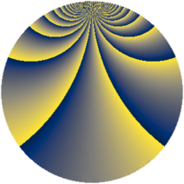# Properties

 Label 429.2.bvLevel $429$ Weight $2$ Character orbit 429.bv Rep. character $\chi_{429}(20,\cdot)$ Character field $\Q(\zeta_{60})$ Dimension $832$ Newform subspaces $1$ Sturm bound $112$ Trace bound $0$

# Related objects

## Defining parameters

 Level: $$N$$ $$=$$ $$429 = 3 \cdot 11 \cdot 13$$ Weight: $$k$$ $$=$$ $$2$$ Character orbit: $$[\chi]$$ $$=$$ 429.bv (of order $$60$$ and degree $$16$$) Character conductor: $$\operatorname{cond}(\chi)$$ $$=$$ $$429$$ Character field: $$\Q(\zeta_{60})$$ Newform subspaces: $$1$$ Sturm bound: $$112$$ Trace bound: $$0$$

## Dimensions

The following table gives the dimensions of various subspaces of $$M_{2}(429, [\chi])$$.

Total New Old
Modular forms 960 960 0
Cusp forms 832 832 0
Eisenstein series 128 128 0

## Trace form

 $$832q - 6q^{3} - 36q^{4} - 4q^{6} - 24q^{7} - 6q^{9} + O(q^{10})$$ $$832q - 6q^{3} - 36q^{4} - 4q^{6} - 24q^{7} - 6q^{9} - 96q^{10} - 40q^{13} + 12q^{15} - 68q^{16} - 20q^{18} - 32q^{19} - 84q^{21} - 24q^{22} - 72q^{24} - 114q^{30} - 8q^{31} + 14q^{33} - 24q^{34} + 78q^{36} - 24q^{37} - 72q^{39} - 112q^{40} + 2q^{42} - 96q^{43} + 4q^{45} - 16q^{46} + 14q^{48} - 36q^{49} + 8q^{52} + 32q^{54} - 24q^{55} - 12q^{57} + 112q^{58} - 120q^{60} + 12q^{61} + 28q^{63} - 300q^{66} - 32q^{67} - 18q^{69} - 140q^{70} + 204q^{72} - 24q^{73} + 66q^{75} + 176q^{76} - 28q^{78} - 224q^{79} - 58q^{81} - 264q^{82} + 20q^{84} - 168q^{85} + 72q^{87} - 120q^{88} - 146q^{93} - 4q^{94} - 10q^{96} - 88q^{97} + 40q^{99} + O(q^{100})$$

## Decomposition of $$S_{2}^{\mathrm{new}}(429, [\chi])$$ into newform subspaces

Label Dim. $$A$$ Field CM Traces $q$-expansion
$$a_2$$ $$a_3$$ $$a_5$$ $$a_7$$
429.2.bv.a $$832$$ $$3.426$$ None $$0$$ $$-6$$ $$0$$ $$-24$$